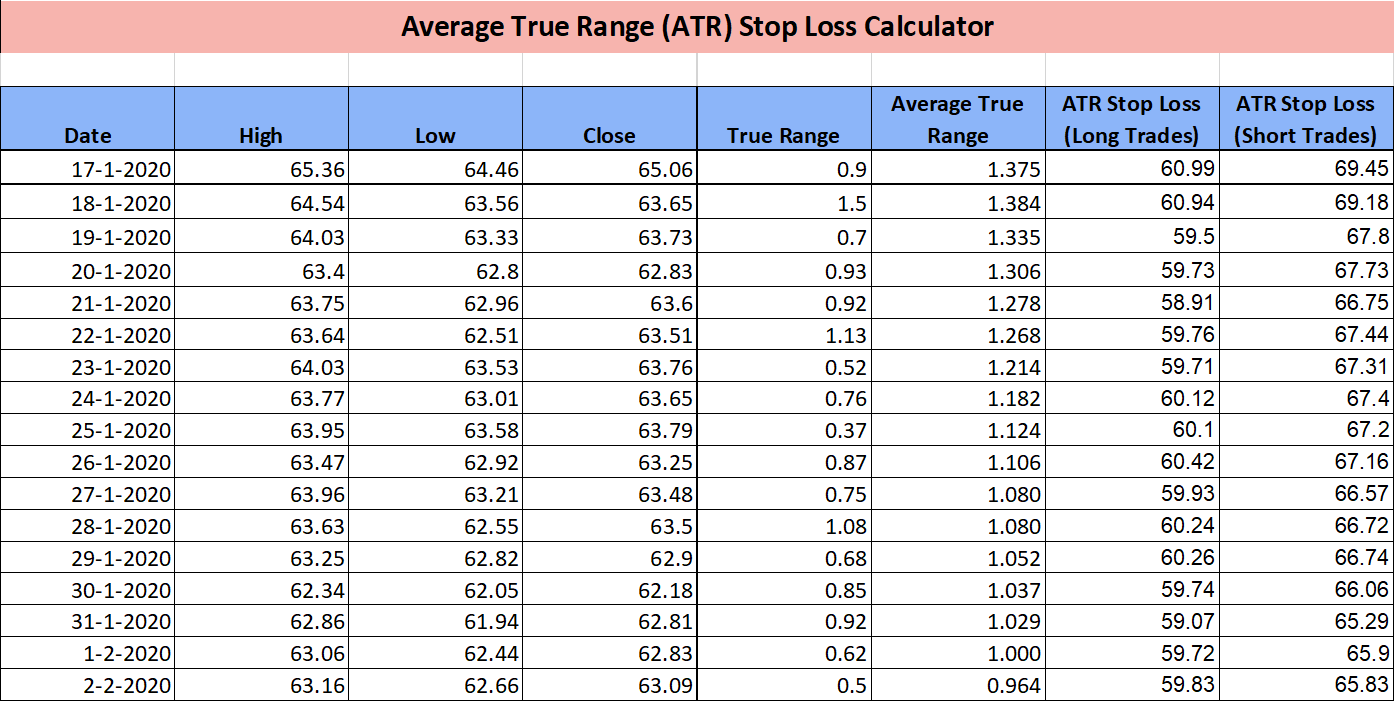# Calculating ATR based Stop Loss in an Excel Sheet

There is no denial of the fact that Stop Loss is an integral part of any trading strategy. Trading without a stop loss is analogous to jumping off the plane without a parachute. The most common stop loss strategy is a fixed stop loss, but it may not be the best one. In this article, we are going to discuss a better way of managing stop losses using the average true range (ATR). Also, we’ll understand how to calculate ATR based stop loss in an Excel sheet.

## Problems with Standard Stop Loss

The biggest disadvantage of a standard stoploss is that it is fixed. 99% of people use fixed stoploss in terms of percentage of points. For example – enter a trade and apply a stoploss of 2%.

While standard stop loss works in a non-volatile market, there is a very high probability that your stop loss gets hit and again the price reverses on occasions when volatility is high.

I am sure most of us have got into this trap of stop-loss hunting and lost a lot of money.

## How ATR Stop Loss solves these Problems?

ATR based stop-loss strategy adapts to changing market conditions. When volatility is high, traders use a larger stop loss to account for greater market swings. When volatility is low, traders use a more conservative stop loss.

The calculation of stop-loss, in this case, is based on the Average True Range (ATR) indicator. We’ll discuss the calculation steps in the next section

ATR is the standard measure of volatility for a given time period. The most common time period used for ATR calculation is 14.

If the ATR is high the market is more volatile, while a lower ATR indicates a less volatile market. Using ATR makes stop-loss dynamic so that it adapts to the market conditions and you don’t get false stopped out.

## How to Calculate ATR based Stop Loss in an Excel Sheet?

ATR is calculated based on True Range (TR).

True range is the largest of:

1. (Current High – Current Low)
2. (Current High – Previous Close)
3. (Current Low – Previous Close)

Absolute values are used in case the result is negative.

ATR is then calculated using the below formula:

Current ATR = [(Prior ATR x (n-1)) + Current TR] / n

where n = time period of ATR

Since there must be a beginning, the first TR value is simply the High minus the Low, and the first n-day ATR is the average of the daily TR values for the last n days.

We’ve incorporated these calculations already in the ATR excel sheet. See screenshot below:For long trades, ATR*3 is subtracted from the Close price to compute stop loss. And for short trades, ATR*3 is added to the close price. 3 is a common multiplier, but you may also try 2 as a multiplier to tighten your stop loss.

And ATR is added/subtracted from close price just for the illustration. In the ideal case, you must apply ATR based stop loss on your trade entry price.

## How to use this Excel Sheet?

It’s fairly simple to use this excel sheet.

You just need to enter High, Low, and Close price with dates in the first 4 columns of the sheet and everything else would be calculated automatically.

Make sure you have atleast 14 data points, as that is the bare minimum requirement to calculate 14-period ATR.

Once the ATR is calculated, you can use the previous day’s ATR value to compute stop loss for the current day.

ATR Stop Loss Calculator Excel Sheet

Hope you liked the post. Please let us know if you have any questions about calculating ATR based Stop Loss in an Excel Sheet.### One Comment

1.Nitil

Thank you for your tutorial on ATR trailing stop loss calculation. Can I further request you to help me on calculation of ATR trailing stop loss for lower time frame i.e. 3 min, 5 min, 15 min, etc. for Intraday perspective.
Regards,
Nitil